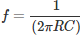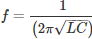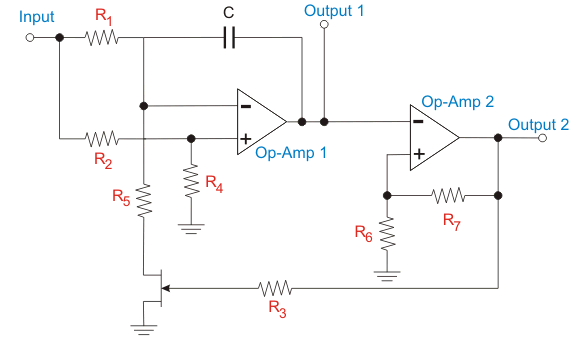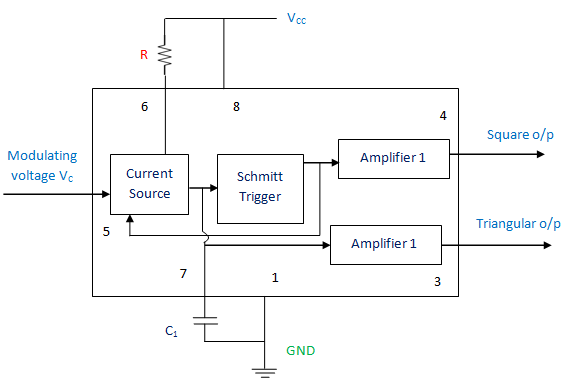# Voltage Controlled Oscillator | VCO

Voltage controlled oscillator (VCO), from the name itself it is clear that the output instantaneous frequency of the oscillator is controlled by the input voltage. It is a kind of oscillator which can produce output signal frequency over a large range (few Hertz-hundreds of Giga Hertz) depending on the input DC voltage given to it.

## Frequency Control in Voltage Controlled Oscillator

Many forms of VCOs are generally used. It can be of RC oscillator or multi vibrator type or LC or crystal oscillator type. However; if it is of RC oscillator type, the oscillation frequency of output signal will be in inversely proportional to capacitance asIn the case of LC oscillator, the oscillation frequency of output signal will beSo, we can say that as the input voltage or control voltage increases, the capacitance get reduced. Hence, the control voltage and frequency of oscillations are directly proportional. That is, when one increases, the other will increase.The figure above represents the basic working of voltage controlled oscillator. Here, we can see that at nominal control voltage represented by VC(nom), the oscillator works at its free running or normal frequency, fC(nom). As the control voltage decreases from nominal voltage, the frequency also decreases and as the nominal control voltage increases, the frequency also gets higher.
The varactors diodes which are variable capacitance diodes (available in different capacitance range) are implemented for getting this variable voltage. For low frequency oscillators, the charging rate of capacitors is altered using voltage controlled current source to get the variable voltage.

## Types of Voltage Controlled Oscillator

The VCOs can be categorized based on the output waveform:

• Harmonic Oscillators
• Relaxation Oscillators

### Harmonic Oscillators

The output waveform produced by harmonic oscillators is sinusoidal. This can often referred as linear voltage controlled oscillator. The examples are LC and Crystal oscillators. Here, the capacitance of the varactor diode is varied by the voltage which is across the diode. This in turns alters the capacitance of the LC circuit. Hence, the output frequency will change. Advantages are frequency stability with reference to power supply, noise and temperature, Accuracy in control of frequency. The main drawback is this type of oscillators cannot be implemented effortlessly on monolithic ICs.

### Relaxation Oscillators

The output waveform produced by harmonic oscillators is saw tooth. This type can give a large range of frequency using reduced quantity of components. Mainly it can be used in monolithic ICs. The relaxation oscillators can possess the following topologies:

• Delay-based ring VCOs
• Grounded capacitor VCOs
• Emitter-Coupled VCOs

Here; in delay-based ring VCOs, the gain stages are attached together in a ring form. As the name implies, the frequency is related to the delay in every single stage. The second and third type VCOs works almost similarly. The time period taken in each stage is directly related to the charging and discharging time of capacitor.

## Working Principle of Voltage Controlled Oscillator (VCO)

VCO circuits can be designed by means of many voltage control electronic components such as varactor diodes, transistors, Op-amps etc. Here, we are going to discuss about the working of a VCO using Op-amps. The circuit diagram is shown below.The output waveform of this VCO will be square wave. As we know the output frequency is related to the control voltage. In this circuit the first Op-amp will function as an integrator. The voltage divider arrangement is implemented here. Because of this, the half of the control voltage that is given as input is given to the positive terminal of the Op-amp 1. The same level of voltage is maintained at the negative terminal. This is to sustain the voltage drop across the resistor, R1 as half of the control voltage.
When the MOSFET is in on condition, the current flowing from the R1 resistor passes through the MOSFET. The R2 have half the resistance, same voltage drop and twice the current as that of R1. So, the extra current charges the connected capacitor. The Op-amp 1 should provide a gradually increasing output voltage to supply this current.
When the MOSFET is in off condition, the current flowing from the R1 resistor passes through the capacitor, get discharged. The output voltage obtained from the Op-amp 1 at this time will be falling. As a result, a triangular waveform is generated as the output of Op-amp 1.
The Op-amp 2 will operate as Schmitt trigger. The input to this Op-amp is triangular wave which is the output of the Op-amp 1. If the input voltage is higher than the threshold level, the output from the Op-amp 2 will be VCC. If the input voltage is less than the threshold level, the output from the Op-amp 2 will be zero. Therefore, the output of the Op-amp 2 will be square wave.
Example of VCO is LM566 IC or IC 566. It is in fact an 8 pin integrated circuit which can produce double outputs-square wave and triangular wave. The internal circuit is represented below.## Applications of Voltage Controlled Oscillator

• Function generator
• Phase Locked Loop
• Tone generator
• Frequency-shift keying
• Frequency modulation
Want To Learn Faster? 🎓
Get electrical articles delivered to your inbox every week.
No credit card required—it’s 100% free.